# SSAT Upper Level Math : How to solve arithmetic word problems

## Example Questions

← Previous 1

### Example Question #1 : How To Solve Arithmetic Word Problems

What is the ratio of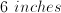to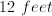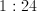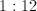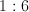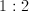Explanation:

First, we need to convert 6 inches into feet.

There are 12 inches in a foot.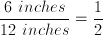.

So 6 inches is equal to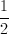feet.  Now our ratio isto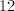.  To find this ratio we divide 12 by.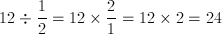.

So the ratio is 1:24.

### Example Question #2 : Arithmetic Word Problems

What is the ratio of 3 gallons to 5 quarts?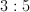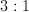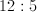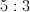Explanation:

First convert gallons to quarts.  There are 4 quarts in a gallon.  So 3 gallons is equivalent to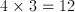quarts.

Now we have 12 quarts to 5 quarts.  This ratio cannot reduce, so our answer is 12:5.

### Example Question #7 : Word Problems

Bob gets paid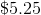an hour for the regular hours he works and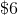an hour for any overtime hours he works. All hours over 40 in a week are considered overtime. If Bob works 44 hours this week, how much did he make?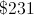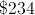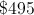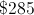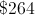Explanation:

You first calculate how much he makes for normal hours, which is the number of hours works multiplied by the wage. So for normal hours,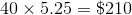. For overtime, it is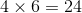. Add the amount made in overtime and the normal hours and you get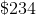.

### Example Question #1 : How To Solve Arithmetic Word Problems

There are 500 students at the high school. There are only two menu options: chicken or fish. If 15% of students ordered fish, how many students ordered chicken?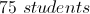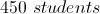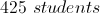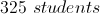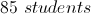Explanation:

If 15% of students ordered fish, then 85% of the students must have ordered chicken. Then multiply 85% or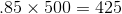.

### Example Question #1 : How To Do Distance Problems

1 mile = 5280 feet

If Greg's house is 5.3 miles away, how far is it in feet?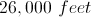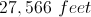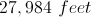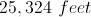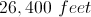Explanation:

Using the conversion formula, you would multiply 5.3 miles by 5280 feet and you will get 27,984 feet.

### Example Question #2 : How To Solve Arithmetic Word Problems

Convert 15.68 to a percent.

1568%

15.68%

1.568%

15680%

156.8%

1568%

Explanation:

To convert a number to a percent, you just multiply it by. So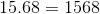%.

### Example Question #3 : How To Solve Arithmetic Word Problems

What number can 1536 be divided by that would give no remainder?Explanation: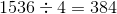### Example Question #4 : How To Solve Arithmetic Word Problems

Candidate A getsof the votes in an election.

Candidate B gets 35% of the votes.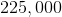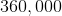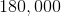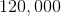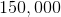Explanation:

First we need to getand 35% in the same terms so that we can subtract one from the other. Since 7ths are repeating decimals, it will be easiest to use fractions.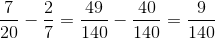.

Now we multiply this fraction by 2.8 million, which reduces nicely to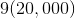, which equals 180,000.

### Example Question #2 : How To Multiply And Divide Fractions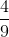of a class of 36 students are boys. If 2 girls and 4 boys were to drop the class, what percentage of the class would be girls?

70%

65%

55%

60%

75%

60%

Explanation:

First determine how many boys and girls are currently in the class.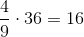boys in the class, which means that there are 20 girls.

When the 6 students drop the new class size will be 30, which will be made up of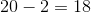girls and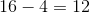boys.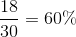### Example Question #1 : How To Solve Arithmetic Word Problems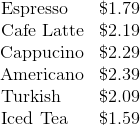Sandy orders some drinks for herself and some friends. She orders three cappuccinos, two iced teas, two cafe lattes, and an espresso. The sales tax is five percent. How much change does she receive back for a twenty-dollar bill?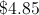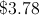A twenty-dollar bill isn't enough to buy the drinks.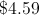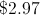Explanation:

The three cappuccinos cost: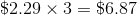The two iced teas cost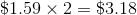The two cafe lattes cost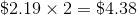The espresso costs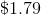.

Add these amounts to get the cost before tax: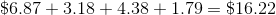The tax is five percent of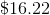or: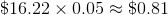Add both values in order to obtain the total cost after tax.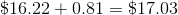As a result, the change from a twenty-dollar bill is as follows: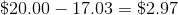← Previous 1